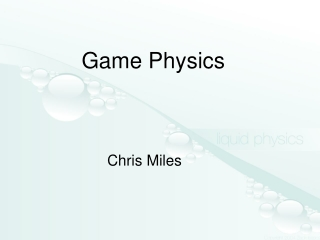DownloadDownload PresentationGame Physics

Game Physics

Download PresentationGame Physics

- - - - - - - - - - - - - - - - - - - - - - - - - - - E N D - - - - - - - - - - - - - - - - - - - - - - - - - - -
Presentation Transcript

1. Game Physics Chris Miles

2. The Goal • To learn how to create game objects with realistic physics models • To learn how to simulate aspects of reality in order to make them behave more realistically

3. Overview • Newton’s laws • Data types • Particle implementation • Rigid body implementation

4. Newton’s Laws of Motion • Inertia • F = ma • Equal and opposite

5. How They Apply • Velocity remains constant unless acted on by a force. • Objects accelerate via f = ma • Interactions between objects should affect both similarly

6. How They Don’t Apply • Velocities that reduce over time give better numerical stability • Objects are often moved instantaneously • Many interactions are one-sided, there is no reason to simulate the other side.

7. An Object – Rigid Body • An object that moves but does not change shape • “Mass properties” define how these objects are affected by forces • Mass • Moment of inertia • Center of gravity

8. Simulation • We want to write a simulation describing the motion of the object • look at the universe after 1 second, 2 seconds, so on and so on. • integrate the equations of motion between those steps

9. Data Types • Scalar • Vector • Matrix • Quaternion • Euler Angles • Rotation Matrix • Tensor

10. Scalars • Scalars are a single number • They represent simple characteristics, such as mass

11. Vectors • [2,5] [1,5,17] [-.14,5,-7] • Vectors usually represent a location, both in world and local space

12. Quaternion • Quaternion’s contain an orientation • Explaining how they work is long and fruitless • Know that they hold an orientation in some undecipherable way • Can quickly rotate vectors, cumulate easily, and interpolate smoothly • Used by virtually every modern game engine (Laura Croft gets points for pioneering)

13. Particles • First we will look at particles, which are a reduced form of Rigid Bodies • Particles differ in that they do not have orientation, they just have positions

14. Euler angles • What quaternions were developed to replace • Vector containing rotations around x,y,z • Suffers from gimbal lock - makes it impossible to go from some orientations to others in a single step • Interpolates poorly - very ugly animations / camera movement • Understandable

15. Rotation matrixes • Another alternative to quaternions • Too large, requires 9 variables vs. 4 • Numerically unstable – unnormalizes over time • Slower

16. Tensor • Don’t worry about them, math term • Generalization of scalars/vectors/matrix’s • Scalars are rank 0 tensor, vectors 1, matrix’s 2 • Concerned with rank 2 tensors, for moment of inertia • Can also accurately simulate drag

17. Particle Class Definition • Class Particle • vector position • vector velocity • vector resultantForce • scalar mass • Function Integrate( dtime ) • Called every time step to update position

18. Function Integrate( dtime ) //dtime = how much time has passed in this time step position += velocity * dtime velocity += force / mass force = 0

19. Improvements • Violate law #1, have velocities slowly decrease – greatly increases numerical stability • Original: • velocity += force / mass * dtime • New: • velocity += (force/mass – velocity*cf)* dtime • cf should be a coefficient of on the order of .999 or so

20. Implicit Integration • We are doing explicit (forward) euler integration so far in our modeling. • If stability is an issue you can use implicit integration • http://www.mech.gla.ac.uk/~peterg/software/MTT/examples/Simulation_rep/node89.html is a good overview

21. Runge-Kutta • Precise integration technique • Sample several times for each time step. • t = 0, t = .25, t = .5, t = .75 to find value at t = 1 • Provides very accurate results • http://mathworld.wolfram.com/Runge-KuttaMethod.html

22. Rigid Body • Position and Orientation • Linear and Angular movements are independent and analagous

23. Rigid Body Class Definition • Class RigidBody • vector position • vector velocity • vector resultantForce • scalar mass • quaternion orientation • vector rotationalVelocity • vector resultantTorque • tensor momentOfInertia • Function Integrate( dtime )

24. Moments of Inertia • The moment of inertia is the rotational equivalent to mass, it describes how hard an object is to rotate • Depends on the axis of rotation so it is a tensor, mapping from a direction to a magnitude • 3x3 matrix • Just using a simple scalar in this example7

25. Calculating Moments of Inertia • Producing the values that go inside the moment of inertia is non trivial. • Assign point masses to the object, representing where things are distributed • Then Σmr2

26. Ideal Integrate function position += velocity * dtime velocity += force / mass * dtime force = 0 orientation += rotationalVelocity * dtime rotationalVelocity += torque / momentOfInertia * dtime torque = 0

27. Complications • Quaternion operators are odd so to add rotationalVelocity • from • orientation += rotationalVelocity * dtime • to • o += (rv * o) / 2.0f * dtime;

28. Point Forces • To calculate the torque caused by point forces • Use the vector cross product • force X position = torque • Where position is the vector from the center of gravity to the point